Anúncio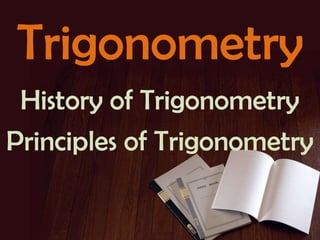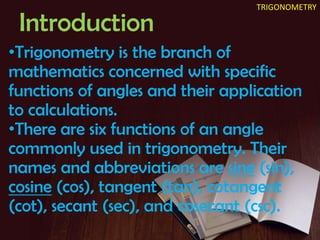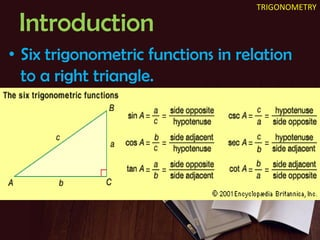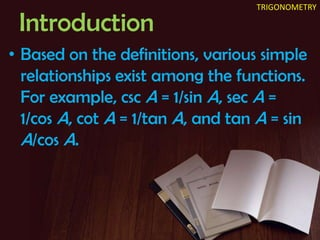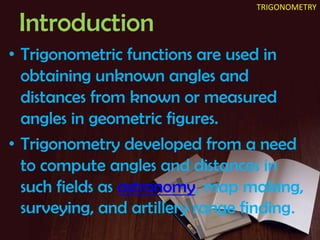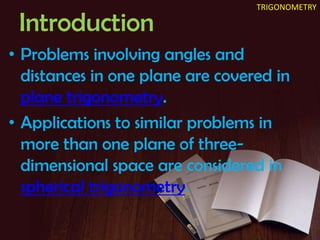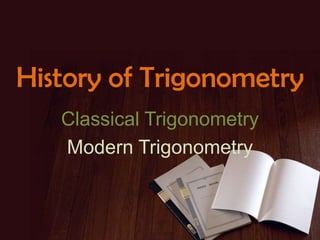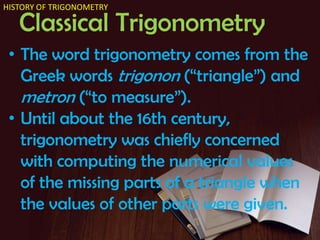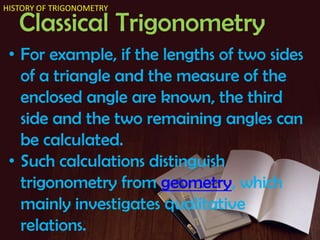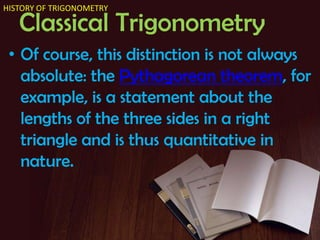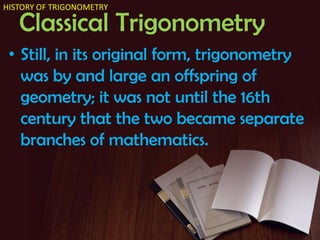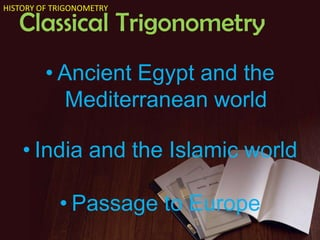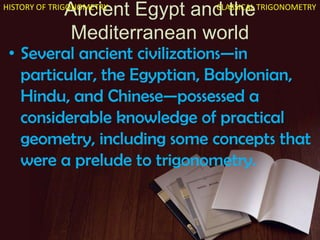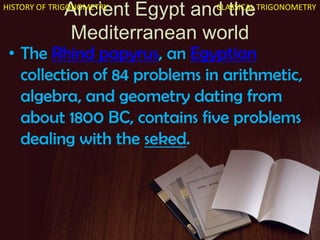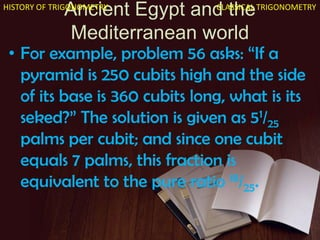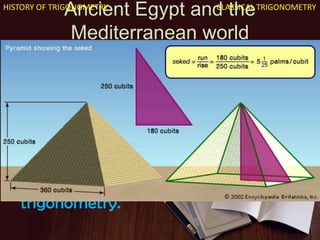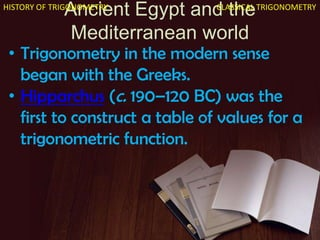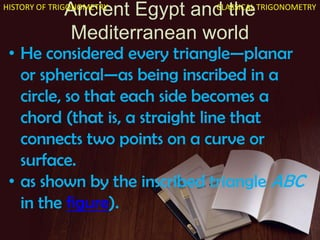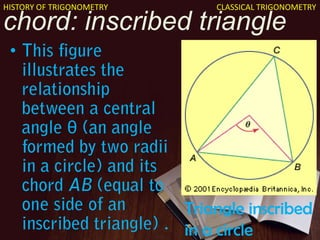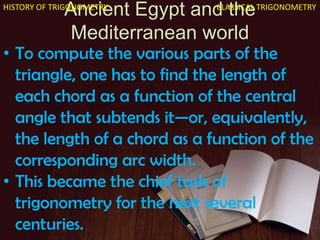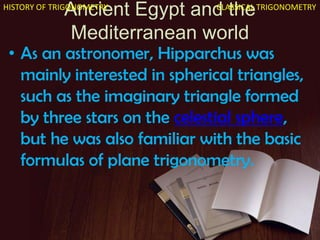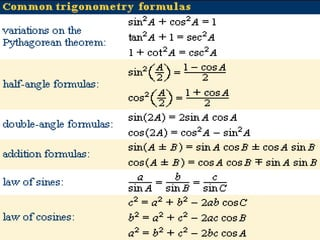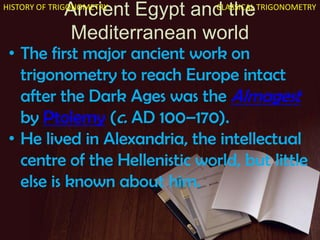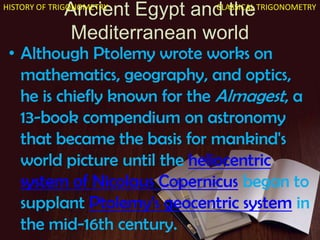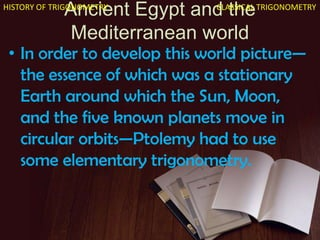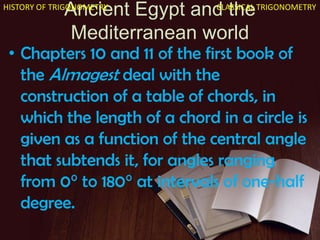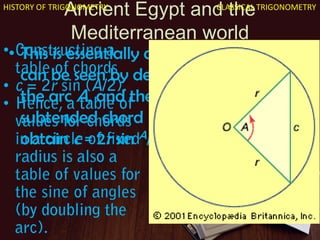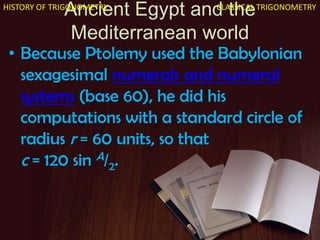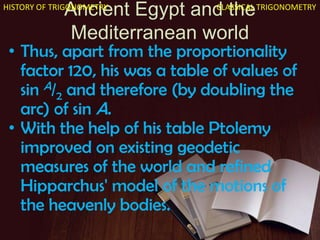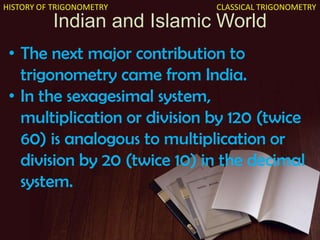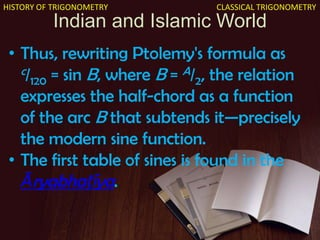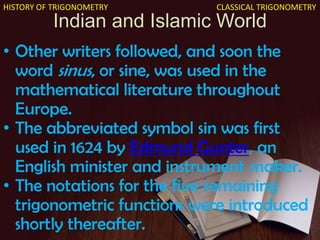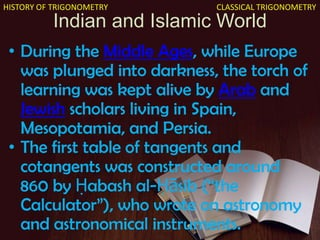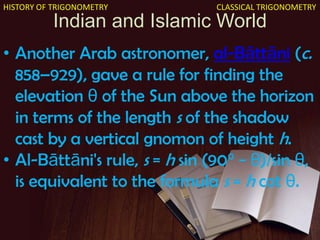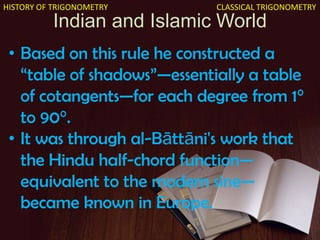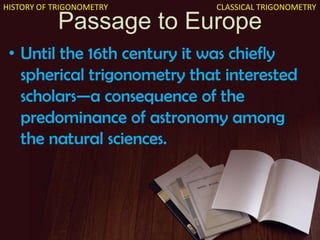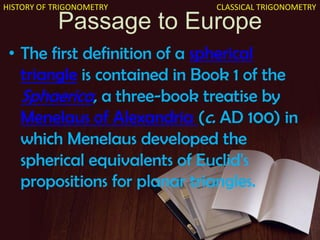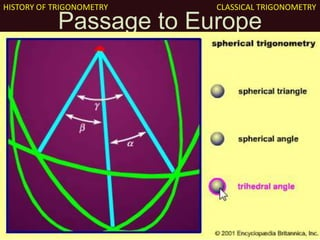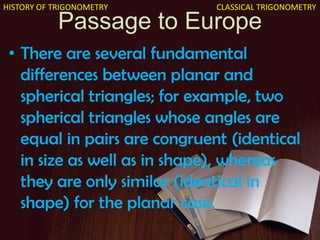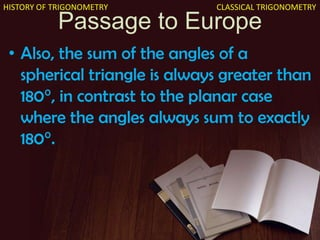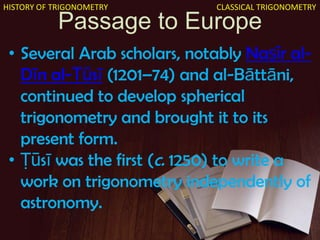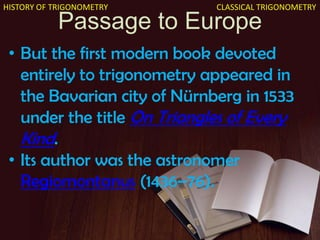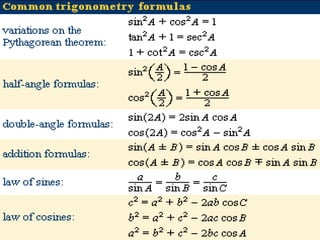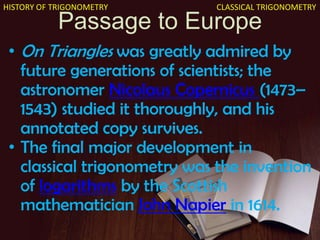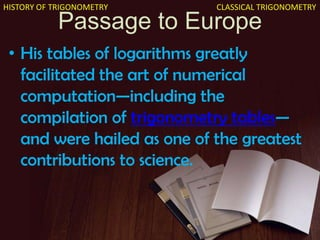1 de 50
Anúncio

### History of trigonometry clasical - animated

1. Trigonometry History of Trigonometry Principles of Trigonometry
2. Introduction •Trigonometry is the branch of mathematics concerned with specific functions of angles and their application to calculations. •There are six functions of an angle commonly used in trigonometry. Their names and abbreviations are sine (sin), cosine (cos), tangent (tan), cotangent (cot), secant (sec), and cosecant (csc). TRIGONOMETRY
3. Introduction • Six trigonometric functions in relation to a right triangle. TRIGONOMETRY
4. Introduction • Based on the definitions, various simple relationships exist among the functions. For example, csc A = 1/sin A, sec A = 1/cos A, cot A = 1/tan A, and tan A = sin A/cos A. TRIGONOMETRY
5. Introduction • Trigonometric functions are used in obtaining unknown angles and distances from known or measured angles in geometric figures. • Trigonometry developed from a need to compute angles and distances in such fields as astronomy, map making, surveying, and artillery range finding TRIGONOMETRY
6. Introduction • Problems involving angles and distances in one plane are covered in plane trigonometry. • Applications to similar problems in more than one plane of three- dimensional space are considered in spherical trigonometry. TRIGONOMETRY
7. History of Trigonometry Classical Trigonometry Modern Trigonometry
8. Classical Trigonometry • The word trigonometry comes from the Greek words trigonon (―triangle‖) and metron (―to measure‖). • Until about the 16th century, trigonometry was chiefly concerned with computing the numerical values of the missing parts of a triangle when the values of other parts were given. HISTORY OF TRIGONOMETRY
9. Classical Trigonometry • For example, if the lengths of two sides of a triangle and the measure of the enclosed angle are known, the third side and the two remaining angles can be calculated. • Such calculations distinguish trigonometry from geometry, which mainly investigates qualitative relations. HISTORY OF TRIGONOMETRY
10. Classical Trigonometry • Of course, this distinction is not always absolute: the Pythagorean theorem, for example, is a statement about the lengths of the three sides in a right triangle and is thus quantitative in nature. HISTORY OF TRIGONOMETRY
11. Classical Trigonometry • Still, in its original form, trigonometry was by and large an offspring of geometry; it was not until the 16th century that the two became separate branches of mathematics. HISTORY OF TRIGONOMETRY
12. Classical Trigonometry • Ancient Egypt and the Mediterranean world • India and the Islamic world • Passage to Europe HISTORY OF TRIGONOMETRY
13. Ancient Egypt and the Mediterranean world • Several ancient civilizations—in particular, the Egyptian, Babylonian, Hindu, and Chinese—possessed a considerable knowledge of practical geometry, including some concepts that were a prelude to trigonometry. HISTORY OF TRIGONOMETRY CLASSICAL TRIGONOMETRY
14. Ancient Egypt and the Mediterranean world • The Rhind papyrus, an Egyptian collection of 84 problems in arithmetic, algebra, and geometry dating from about 1800 BC, contains five problems dealing with the seked. HISTORY OF TRIGONOMETRY CLASSICAL TRIGONOMETRY
15. Ancient Egypt and the Mediterranean world • For example, problem 56 asks: ―If a pyramid is 250 cubits high and the side of its base is 360 cubits long, what is its seked?‖ The solution is given as 51/25 palms per cubit; and since one cubit equals 7 palms, this fraction is equivalent to the pure ratio 18/25. HISTORY OF TRIGONOMETRY CLASSICAL TRIGONOMETRY
16. Ancient Egypt and the Mediterranean world • This is actually the ―run-to-rise‖ ratio of the pyramid in question—in effect, the cotangent of the angle between the base and face (see the figure). • It shows that the Egyptians had at least some knowledge of the numerical relations in a triangle, a kind of ―proto- trigonometry.‖ HISTORY OF TRIGONOMETRY CLASSICAL TRIGONOMETRY
17. Ancient Egypt and the Mediterranean world • Trigonometry in the modern sense began with the Greeks. • Hipparchus (c. 190–120 BC) was the first to construct a table of values for a trigonometric function. HISTORY OF TRIGONOMETRY CLASSICAL TRIGONOMETRY
18. Ancient Egypt and the Mediterranean world • He considered every triangle—planar or spherical—as being inscribed in a circle, so that each side becomes a chord (that is, a straight line that connects two points on a curve or surface. • as shown by the inscribed triangle ABC in the figure). HISTORY OF TRIGONOMETRY CLASSICAL TRIGONOMETRY
19. chord: inscribed triangle • θ Triangle inscribed in a circle HISTORY OF TRIGONOMETRY CLASSICAL TRIGONOMETRY
20. Ancient Egypt and the Mediterranean world • To compute the various parts of the triangle, one has to find the length of each chord as a function of the central angle that subtends it—or, equivalently, the length of a chord as a function of the corresponding arc width. • This became the chief task of trigonometry for the next several centuries. HISTORY OF TRIGONOMETRY CLASSICAL TRIGONOMETRY
21. Ancient Egypt and the Mediterranean world • As an astronomer, Hipparchus was mainly interested in spherical triangles, such as the imaginary triangle formed by three stars on the celestial sphere, but he was also familiar with the basic formulas of plane trigonometry. HISTORY OF TRIGONOMETRY CLASSICAL TRIGONOMETRY
22. Ancient Egypt and the Mediterranean world • In Hipparchus's time these formulas were expressed in purely geometric terms as relations between the various chords and the angles (or arcs) that subtend them; the modern symbols for the trigonometric functions were not introduced until the 17th century. • (See the table of common trigonometry formulas.) HISTORY OF TRIGONOMETRY CLASSICAL TRIGONOMETRY
23. Ancient Egypt and the Mediterranean world • The first major ancient work on trigonometry to reach Europe intact after the Dark Ages was the Almagest by Ptolemy (c. AD 100–170). • He lived in Alexandria, the intellectual centre of the Hellenistic world, but little else is known about him. HISTORY OF TRIGONOMETRY CLASSICAL TRIGONOMETRY
24. Ancient Egypt and the Mediterranean world • Although Ptolemy wrote works on mathematics, geography, and optics, he is chiefly known for the Almagest, a 13-book compendium on astronomy that became the basis for mankind's world picture until the heliocentric system of Nicolaus Copernicus began to supplant Ptolemy's geocentric system in the mid-16th century. HISTORY OF TRIGONOMETRY CLASSICAL TRIGONOMETRY
25. Ancient Egypt and the Mediterranean world • In order to develop this world picture— the essence of which was a stationary Earth around which the Sun, Moon, and the five known planets move in circular orbits—Ptolemy had to use some elementary trigonometry. HISTORY OF TRIGONOMETRY CLASSICAL TRIGONOMETRY
26. Ancient Egypt and the Mediterranean world • Chapters 10 and 11 of the first book of the Almagest deal with the construction of a table of chords, in which the length of a chord in a circle is given as a function of the central angle that subtends it, for angles ranging from 0° to 180° at intervals of one-half degree. HISTORY OF TRIGONOMETRY CLASSICAL TRIGONOMETRY
27. Ancient Egypt and the Mediterranean world • This is essentially a table of sines, which can be seen by denoting the radius r, the arc A, and the length of the subtended chord c (see the figure), to obtain c = 2r sin A/2. HISTORY OF TRIGONOMETRY CLASSICAL TRIGONOMETRY • • •
28. Ancient Egypt and the Mediterranean world • Because Ptolemy used the Babylonian sexagesimal numerals and numeral systems (base 60), he did his computations with a standard circle of radius r = 60 units, so that c = 120 sin A/2. HISTORY OF TRIGONOMETRY CLASSICAL TRIGONOMETRY
29. Ancient Egypt and the Mediterranean world • Thus, apart from the proportionality factor 120, his was a table of values of sin A/2 and therefore (by doubling the arc) of sin A. • With the help of his table Ptolemy improved on existing geodetic measures of the world and refined Hipparchus' model of the motions of the heavenly bodies. HISTORY OF TRIGONOMETRY CLASSICAL TRIGONOMETRY
30. Classical Trigonometry • Ancient Egypt and the Mediterranean world • India and the Islamic world • Passage to Europe HISTORY OF TRIGONOMETRY
31. Indian and Islamic World • The next major contribution to trigonometry came from India. • In the sexagesimal system, multiplication or division by 120 (twice 60) is analogous to multiplication or division by 20 (twice 10) in the decimal system. HISTORY OF TRIGONOMETRY CLASSICAL TRIGONOMETRY
32. Indian and Islamic World • Thus, rewriting Ptolemy's formula as c/120 = sin B, where B = A/2, the relation expresses the half-chord as a function of the arc B that subtends it—precisely the modern sine function. • The first table of sines is found in the Āryabhaṭīya. HISTORY OF TRIGONOMETRY CLASSICAL TRIGONOMETRY
33. Indian and Islamic World • Its author, Āryabhaṭa I (c. 475–550), used the word ardha-jya for half- chord, which he sometimes turned around to jya-ardha (―chord-half‖); in due time he shortened it to jya or jiva. • Later, when Muslim scholars translated this work into Arabic, they retained the word jiva without translating its meaning. HISTORY OF TRIGONOMETRY CLASSICAL TRIGONOMETRY
34. Indian and Islamic World • In Semitic languages words consist mostly of consonants, the pronunciation of the missing vowels being understood by common usage. • Thus jiva could also be pronounced as jiba or jaib, and this last word in Arabic means ―fold‖ or ―bay.‖ HISTORY OF TRIGONOMETRY CLASSICAL TRIGONOMETRY
35. Indian and Islamic World • When the Arab translation was later translated into Latin, jaib became sinus, the Latin word for bay. • The word sinus first appeared in the writings of Gherardo of Cremona (c. 1114–87), who translated many of the Greek texts, including the Almagest, into Latin. HISTORY OF TRIGONOMETRY CLASSICAL TRIGONOMETRY
36. Indian and Islamic World • Other writers followed, and soon the word sinus, or sine, was used in the mathematical literature throughout Europe. • The abbreviated symbol sin was first used in 1624 by Edmund Gunter, an English minister and instrument maker. • The notations for the five remaining trigonometric functions were introduced shortly thereafter. HISTORY OF TRIGONOMETRY CLASSICAL TRIGONOMETRY
37. Indian and Islamic World HISTORY OF TRIGONOMETRY • During the Middle Ages, while Europe was plunged into darkness, the torch of learning was kept alive by Arab and Jewish scholars living in Spain, Mesopotamia, and Persia. • The first table of tangents and cotangents was constructed around 860 by Ḥabash al-Ḥāsib (―the Calculator‖), who wrote on astronomy and astronomical instruments. CLASSICAL TRIGONOMETRY
38. Indian and Islamic World • Another Arab astronomer, al-Bāttāni (c. 858–929), gave a rule for finding the elevation θ of the Sun above the horizon in terms of the length s of the shadow cast by a vertical gnomon of height h. • Al-Bāttāni's rule, s = h sin (90° − θ)/sin θ, is equivalent to the formula s = h cot θ. HISTORY OF TRIGONOMETRY CLASSICAL TRIGONOMETRY
39. Indian and Islamic World • Based on this rule he constructed a ―table of shadows‖—essentially a table of cotangents—for each degree from 1° to 90°. • It was through al-Bāttāni's work that the Hindu half-chord function— equivalent to the modern sine— became known in Europe. HISTORY OF TRIGONOMETRY CLASSICAL TRIGONOMETRY
40. Classical Trigonometry • Ancient Egypt and the Mediterranean world • India and the Islamic world • Passage to Europe HISTORY OF TRIGONOMETRY
41. Passage to Europe • Until the 16th century it was chiefly spherical trigonometry that interested scholars—a consequence of the predominance of astronomy among the natural sciences. HISTORY OF TRIGONOMETRY CLASSICAL TRIGONOMETRY
42. Passage to Europe • The first definition of a spherical triangle is contained in Book 1 of the Sphaerica, a three-book treatise by Menelaus of Alexandria (c. AD 100) in which Menelaus developed the spherical equivalents of Euclid's propositions for planar triangles. HISTORY OF TRIGONOMETRY CLASSICAL TRIGONOMETRY
43. Passage to Europe • A spherical triangle was understood to mean a figure formed on the surface of a sphere by three arcs of great circles, that is, circles whose centres coincide with the centre of the sphere (as shown in the animation). HISTORY OF TRIGONOMETRY CLASSICAL TRIGONOMETRY
44. Passage to Europe • There are several fundamental differences between planar and spherical triangles; for example, two spherical triangles whose angles are equal in pairs are congruent (identical in size as well as in shape), whereas they are only similar (identical in shape) for the planar case. HISTORY OF TRIGONOMETRY CLASSICAL TRIGONOMETRY
45. Passage to Europe • Also, the sum of the angles of a spherical triangle is always greater than 180°, in contrast to the planar case where the angles always sum to exactly 180°. HISTORY OF TRIGONOMETRY CLASSICAL TRIGONOMETRY
46. Passage to Europe • Several Arab scholars, notably Naṣīr al- Dīn al-Ṭūsī (1201–74) and al-Bāttāni, continued to develop spherical trigonometry and brought it to its present form. • Ṭūsī was the first (c. 1250) to write a work on trigonometry independently of astronomy. HISTORY OF TRIGONOMETRY CLASSICAL TRIGONOMETRY
47. Passage to Europe • But the first modern book devoted entirely to trigonometry appeared in the Bavarian city of Nürnberg in 1533 under the title On Triangles of Every Kind. • Its author was the astronomer Regiomontanus (1436–76). HISTORY OF TRIGONOMETRY CLASSICAL TRIGONOMETRY
48. Passage to Europe • On Triangles contains all the theorems needed to solve triangles, planar or spherical—although these theorems are expressed in verbal form, as symbolic algebra had yet to be invented. • In particular, the law of sines (see the table) is stated in essentially the modern way. HISTORY OF TRIGONOMETRY CLASSICAL TRIGONOMETRY
49. Passage to Europe • On Triangles was greatly admired by future generations of scientists; the astronomer Nicolaus Copernicus (1473– 1543) studied it thoroughly, and his annotated copy survives. • The final major development in classical trigonometry was the invention of logarithms by the Scottish mathematician John Napier in 1614. HISTORY OF TRIGONOMETRY CLASSICAL TRIGONOMETRY
50. Passage to Europe • His tables of logarithms greatly facilitated the art of numerical computation—including the compilation of trigonometry tables— and were hailed as one of the greatest contributions to science. HISTORY OF TRIGONOMETRY CLASSICAL TRIGONOMETRY
Anúncio